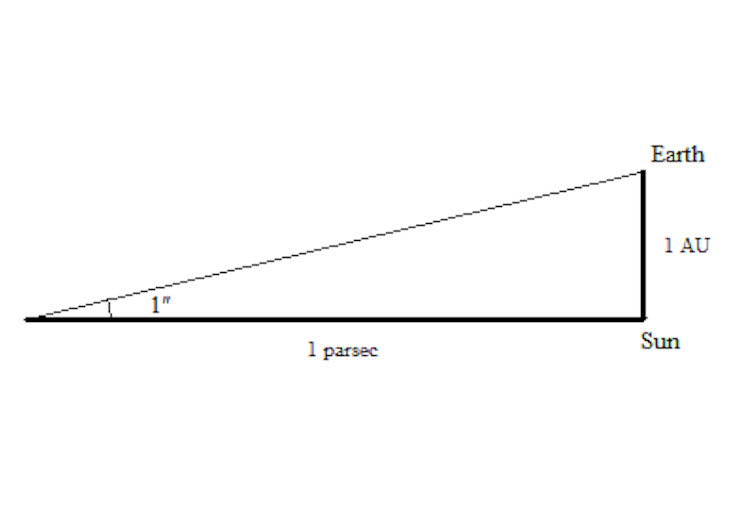How does an astronomical unit differ from a light year?

Jun 14, 2014

A light year (ly) is the distance light travels in a year, while the astronomical unit (AU) is the average observed distance to the sun.

The SI unit system defines the speed of light as roughly $3.00 \setminus \times {10}^{8} \cdot \text{meter"/"second}$. We can use a unit conversion to find the distance light travels in one year.

$3.00 \setminus \times {10}^{8} \cdot \text{meter"/"second"" x "3.16\times10^7 "second"/"year""= "9.42\times10^15"meter"/"year}$

Notice the seconds cancel, leaving the distance light travels in a year. So a light year is roughly $9.42 \setminus \times {10}^{15}$ meters or $5.85 \setminus \times {10}^{12}$ miles.

The average distance from the earth to the sun has been observed to be $1.50 \setminus \times {10}^{11}$ meters or $9.30 \setminus \times {10}^{7}$ miles. That would mean there are approximately $6.32 \setminus \times {10}^{4}$ AUs in one light year.

The AU was first calculated by averaging the maximum and minimal distance to the sun in a year. The aphelion and perihelion respectively.Now a more accurate average is measured. The AU is a great unit to measure distances inside our own solar system, but is impractical over larger distances. For instance our closes celestial neighbor Proxima Centauri is about 4.24 light years away. This would be $2.68 \setminus \times {10}^{5}$ AU.

More on the AU:
The AU is the basis for a larger astronomical unit often used in comparison with light years. The parsec is derived from the AU by a trigonomic relation.